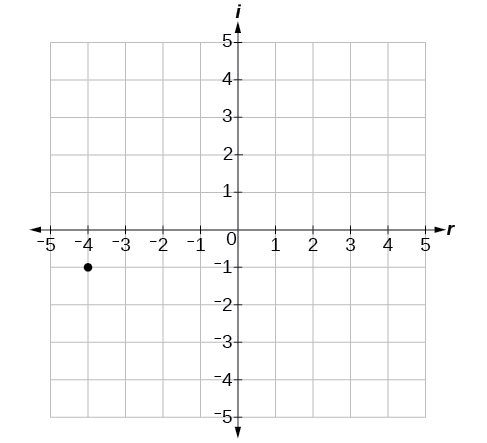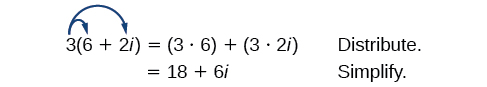# 2.4 Complex numbers  (Page 2/8)

 Page 2 / 8

Let’s consider the number $\text{\hspace{0.17em}}-2+3i.\text{\hspace{0.17em}}$ The real part of the complex number is $\text{\hspace{0.17em}}-2\text{\hspace{0.17em}}$ and the imaginary part is 3. We plot the ordered pair $\text{\hspace{0.17em}}\left(-2,3\right)\text{\hspace{0.17em}}$ to represent the complex number $\text{\hspace{0.17em}}-2+3i,$ as shown in [link] .

## Complex plane

In the complex plane, the horizontal axis is the real axis, and the vertical axis is the imaginary axis, as shown in [link] .

Given a complex number, represent its components on the complex plane.

1. Determine the real part and the imaginary part of the complex number.
2. Move along the horizontal axis to show the real part of the number.
3. Move parallel to the vertical axis to show the imaginary part of the number.
4. Plot the point.

## Plotting a complex number on the complex plane

Plot the complex number $\text{\hspace{0.17em}}3-4i\text{\hspace{0.17em}}$ on the complex plane.

The real part of the complex number is $\text{\hspace{0.17em}}3,$ and the imaginary part is –4. We plot the ordered pair $\text{\hspace{0.17em}}\left(3,-4\right)\text{\hspace{0.17em}}$ as shown in [link] .

Plot the complex number $\text{\hspace{0.17em}}-4-i\text{\hspace{0.17em}}$ on the complex plane.## Adding and subtracting complex numbers

Just as with real numbers, we can perform arithmetic operations on complex numbers. To add or subtract complex numbers, we combine the real parts and then combine the imaginary parts.

## Complex numbers: addition and subtraction

$\left(a+bi\right)+\left(c+di\right)=\left(a+c\right)+\left(b+d\right)i$

Subtracting complex numbers:

$\left(a+bi\right)-\left(c+di\right)=\left(a-c\right)+\left(b-d\right)i$

Given two complex numbers, find the sum or difference.

1. Identify the real and imaginary parts of each number.
2. Add or subtract the real parts.
3. Add or subtract the imaginary parts.

## Adding and subtracting complex numbers

1. $\left(3-4i\right)+\left(2+5i\right)$
2. $\left(-5+7i\right)-\left(-11+2i\right)$

1. $\begin{array}{ccc}\hfill \left(3-4i\right)+\left(2+5i\right)& =& 3-4i+2+5i\hfill \\ & =& 3+2+\left(-4i\right)+5i\hfill \\ & =& \left(3+2\right)+\left(-4+5\right)i\hfill \\ & =& 5+i\hfill \end{array}$

2. $\begin{array}{ccc}\hfill \left(-5+7i\right)-\left(-11+2i\right)& =& -5+7i+11-2i\hfill \\ & =& -5+11+7i-2i\hfill \\ & =& \left(-5+11\right)+\left(7-2\right)i\hfill \\ & =& 6+5i\hfill \end{array}$

Subtract $\text{\hspace{0.17em}}2+5i\text{\hspace{0.17em}}$ from $\text{\hspace{0.17em}}3–4i.$

$\left(3-4i\right)-\left(2+5i\right)=1-9i$

## Multiplying complex numbers

Multiplying complex numbers is much like multiplying binomials. The major difference is that we work with the real and imaginary parts separately.

## Multiplying a complex number by a real number

Lets begin by multiplying a complex number by a real number. We distribute the real number just as we would with a binomial. Consider, for example, $\text{\hspace{0.17em}}3\left(6+2i\right)$ :Given a complex number and a real number, multiply to find the product.

1. Use the distributive property.
2. Simplify.

## Multiplying a complex number by a real number

Find the product $\text{\hspace{0.17em}}4\left(2+5i\right).$

Distribute the 4.

$\begin{array}{ccc}\hfill 4\left(2+5i\right)& =& \left(4\cdot 2\right)+\left(4\cdot 5i\right)\hfill \\ & =& 8+20i\hfill \end{array}$

Find the product: $\text{\hspace{0.17em}}\frac{1}{2}\left(5-2i\right).$

$\frac{5}{2}-i$

## Multiplying complex numbers together

Now, let’s multiply two complex numbers. We can use either the distributive property or more specifically the FOIL method because we are dealing with binomials. Recall that FOIL is an acronym for multiplying First, Inner, Outer, and Last terms together. The difference with complex numbers is that when we get a squared term, $\text{\hspace{0.17em}}{i}^{2},$ it equals $\text{\hspace{0.17em}}-1.$

Given two complex numbers, multiply to find the product.

1. Use the distributive property or the FOIL method.
2. Remember that $\text{\hspace{0.17em}}{i}^{2}=-1.$
3. Group together the real terms and the imaginary terms

root under 3-root under 2 by 5 y square
The sum of the first n terms of a certain series is 2^n-1, Show that , this series is Geometric and Find the formula of the n^th
cosA\1+sinA=secA-tanA
why two x + seven is equal to nineteen.
The numbers cannot be combined with the x
Othman
2x + 7 =19
humberto
2x +7=19. 2x=19 - 7 2x=12 x=6
Yvonne
because x is 6
SAIDI
what is the best practice that will address the issue on this topic? anyone who can help me. i'm working on my action research.
simplify each radical by removing as many factors as possible (a) √75
how is infinity bidder from undefined?
what is the value of x in 4x-2+3
give the complete question
Shanky
4x=3-2 4x=1 x=1+4 x=5 5x
Olaiya
hi can you give another equation I'd like to solve it
Daniel
what is the value of x in 4x-2+3
Olaiya
if 4x-2+3 = 0 then 4x = 2-3 4x = -1 x = -(1÷4) is the answer.
Jacob
4x-2+3 4x=-3+2 4×=-1 4×/4=-1/4
LUTHO
then x=-1/4
LUTHO
4x-2+3 4x=-3+2 4x=-1 4x÷4=-1÷4 x=-1÷4
LUTHO
A research student is working with a culture of bacteria that doubles in size every twenty minutes. The initial population count was  1350  bacteria. Rounding to five significant digits, write an exponential equation representing this situation. To the nearest whole number, what is the population size after  3  hours?
v=lbh calculate the volume if i.l=5cm, b=2cm ,h=3cm
Need help with math
Peya
can you help me on this topic of Geometry if l help you
litshani
( cosec Q _ cot Q ) whole spuare = 1_cosQ / 1+cosQ
A guy wire for a suspension bridge runs from the ground diagonally to the top of the closest pylon to make a triangle. We can use the Pythagorean Theorem to find the length of guy wire needed. The square of the distance between the wire on the ground and the pylon on the ground is 90,000 feet. The square of the height of the pylon is 160,000 feet. So, the length of the guy wire can be found by evaluating √(90000+160000). What is the length of the guy wire?
the indicated sum of a sequence is known as
how do I attempted a trig number as a starter
cos 18 ____ sin 72 evaluateByByBy Vongkol HENGBy Richley CrapoBy OpenStaxBy Janet ForresterBy Ann SchlosserBy Rohini AjayBy Tod McGrathBy OpenStaxBy OpenStaxBy OpenStax